•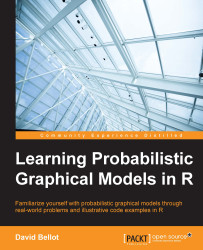#### Learning Probabilistic Graphical Models in R#### Overview of this book

Probabilistic graphical models (PGM, also known as graphical models) are a marriage between probability theory and graph theory. Generally, PGMs use a graph-based representation. Two branches of graphical representations of distributions are commonly used, namely Bayesian networks and Markov networks. R has many packages to implement graphical models. We’ll start by showing you how to transform a classical statistical model into a modern PGM and then look at how to do exact inference in graphical models. Proceeding, we’ll introduce you to many modern R packages that will help you to perform inference on the models. We will then run a Bayesian linear regression and you’ll see the advantage of going probabilistic when you want to do prediction. Next, you’ll master using R packages and implementing its techniques. Finally, you’ll be presented with machine learning applications that have a direct impact in many fields. Here, we’ll cover clustering and the discovery of hidden information in big data, as well as two important methods, PCA and ICA, to reduce the size of big problems.
Learning Probabilistic Graphical Models in RCreditswww.PacktPub.comPrefaceFree Chapter
Probabilistic ReasoningExact InferenceLearning ParametersBayesian Modeling – Basic ModelsApproximate InferenceBayesian Modeling – Linear ModelsProbabilistic Mixture ModelsAppendixIndex## Rejection sampling

Suppose we want to sample from a distribution that is not a simple one. Let's call this distribution p(x) and let's assume we can evaluate p(x) for any given value x, up to a normalizing constant Z, that is: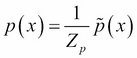In this context, p(x) is too complex to sample from but we have another simpler distribution q(x) from which we can draw samples. Next, we assume there exists a constant k such that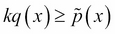for all values of x. The function kq(x) is the comparison function as shown in the following figure: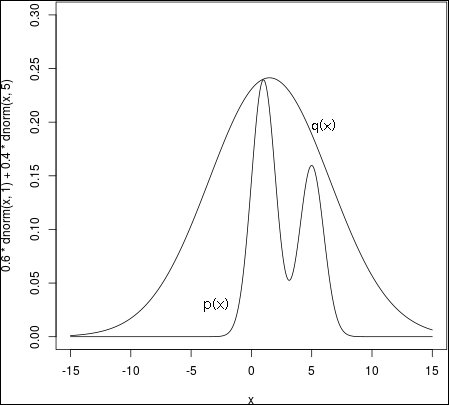The distribution p(x) has been generated with a simple plot:

```0.6*dnorm(x,1)+0.4*dnorm(x,5)
```

The rejection sampling algorithm is based on the following idea:

• Draw a sample z0 from q(z), the proposal distribution

• Draw a second u0 sample from a uniform distribution on [0, kq(z0)]
• If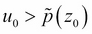then the sample is rejected otherwise u0 is accepted

In the following figure, the pair (z0, u0) is rejected if it lies in the gray area. The accepted pairs are a uniform distribution under the curve of p(z) and therefore...